Courses

# Test: Real Numbers - 3

## 25 Questions MCQ Test Mathematics (Maths) Class 10 | Test: Real Numbers - 3

Description
This mock test of Test: Real Numbers - 3 for Class 10 helps you for every Class 10 entrance exam. This contains 25 Multiple Choice Questions for Class 10 Test: Real Numbers - 3 (mcq) to study with solutions a complete question bank. The solved questions answers in this Test: Real Numbers - 3 quiz give you a good mix of easy questions and tough questions. Class 10 students definitely take this Test: Real Numbers - 3 exercise for a better result in the exam. You can find other Test: Real Numbers - 3 extra questions, long questions & short questions for Class 10 on EduRev as well by searching above.
QUESTION: 1

### Pairs of natural numbers whose least common multiple is 78 and the greatest common divisor is 13 are:

Solution:

Let the no.s be a & b,

GCD [ a , b ] = 13;
=> Let a = 13m and b = 13n

Now, LCM [ a , b ] = 78
=> 13m | 78
=> m | 6 and similarly, n | 6; --> Also, m does not divide n

.'. Possible values of (m,n) are :->
--> [ 1 , 6 ] , [ 2 , 3 ]
.'. Possible values for ( a , b ) are :-> [ 13 , 78 ] , [ 26 , 39 ]

QUESTION: 2

### 1/√3 is -

Solution:

Let as assume to the contrary that 1/✓3 is rational number. Then, we can find coprime integers a and b (≠ 0) such that :
1/✓3= P/Q { where p and Q are co-prime and Q not equal to 0}
✓3 P =Q .1
✓3 = Q/P

Since Q/P is a Rational number

Since L.H.S = R.H.S

So ✓3 is also rational number.

But this contradicts the fact that ✓3 is an irrational number. This contradiction has arisen because of our incorrect assumption that 1/√3 is a rational number.

So this proves that 1/√3 is an irrational number.

QUESTION: 3

### 7√3 is -

Solution:

√3 cannot be expressed in the form p/q where q ≠ 0 which is the form of a rational number. So any number multiplied by it will give an irrational number. So 7√3 is irrational.

QUESTION: 4

5√3 is -

Solution:

To prove : 5-√3 is irrational

Proof:
Let us assume that 5 -√3 is rational.

Let ,
5 - √3 = r , where "r" is rational

5 - r = √3
Here,
LHS is purely rational.But,on the other hand ,RHS is irrational.
Hence,5-√3 is irrational.

QUESTION: 5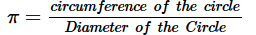Solution:
QUESTION: 6

HCF (p,q) × LCM (p,q) =

Solution:

P=a²b³

q=a³b

HCF (p,q) =a²b

LCM (p.q)=a³b³

As we know that HCF(p,q)xLCM(p,q)=a²bxa³b³
=a⁵b⁴ ---------- equation 1

pq=a²b³xa³b
=a⁵b⁴-----------equation 2

from 1 and 2

we can say that HCF(p,q)xLCM(p,q)=pq

QUESTION: 7

HCF (p,q,r) · LCM (p,q,r) =

Solution:

Since HCF(p,q,r)*LCM(p,q,r) is not equal to pq/r, neither it is equal toqr/p and neither is p,q,r. So the correct answer is D . Also, HCF(p,q,r)*LCM(p,q,r) is not equal to p*q*r . This condition only holds for two numbers.

QUESTION: 8

Two natural numbers whose sum is 85 and the least common multiple is 102 are:

Solution:

Prime factorisation of 102 = 2 x 3 x 17.
Prime factorisation of 85 = 5 x 17 = (2+3) x 17.
The two numbers are:
1. 2 x 17 = 34.
2. 3 x 17 =51

QUESTION: 9

0.737373...=

Solution: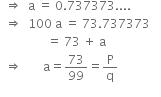⇒ a = 0.737373...
⇒  100a = 73.737373
= 73 + a
⇒ a = 73/99 = p/q
⇒  p=73 and q = 99 are co-prime.
Here, q=3X 11.

QUESTION: 10

If p is a positive prime integer, then √p is –

Solution:

Let us assume, to the contrary, that √p is
rational.
So, we can find coprime integers a and b(b ≠ 0)
such that √p = a/b
⇒ √p b = a
⇒ pb2 = a2 ….(i) [Squaring both the sides]
⇒ a2 is divisible by p
⇒  a is divisible by p
So, we can write a = pc for some integer c.
Therefore, a2 = p2c2 ….[Squaring both the sides]
⇒ pb2 = p2c2 ….[From (i)]
⇒ b2 = pc2
⇒ b2 is divisible by p
⇒ b is divisible by p
⇒ p divides both a and b.
⇒ a and b have at least p as a common factor.
But this contradicts the fact that a and b are coprime.
This contradiction arises because we have
assumed that √p is rational.
Therefore, √p is irrational.

QUESTION: 11

LCM of three numbers 28, 44, 132 is –

Solution:

LCM = 22. 71. 111. 31 = 924

QUESTION: 12

If a is a positive integer and p be a prime number and p divides a2, then

Solution:

If a is a positive integer and p be a prime number and p divides a2

So it implies that p divides a .

QUESTION: 13

If the HCF of 65 and 117 is expressible in the form 65m – 117, then the value of m is

Solution:

Find the HCF of 65 and 117,

117 = 1×65 + 52

65 = 1× 52 + 13

52 = 4 ×13 + 0

∴ HCF of 65 and 117 is 13.

65m - 117 = 13

65m = 117+13 = 130

∴m =130/65 = 2

QUESTION: 14

If x =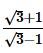and y =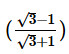, then the value of (x2 + y2) is

Solution:
QUESTION: 15

If x =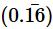, then 3x is –

Solution:
QUESTION: 16

Find the value of x then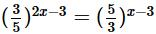Solution:

(3/5)2x-3= (5/3)x-3 (3/5)2x-3=(3/5)3-x 2x-3=3-x 2x+x=6 3x=6 x=2

QUESTION: 17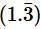is equal to –

Solution:
QUESTION: 18

The product of 4√6 and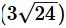is -

Solution: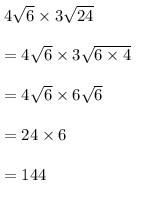QUESTION: 19

If two positive integers a and b are written as a = x3y2 and b = xy3; x, y are prime numbers, then HCF (a, b) is

Solution:

Given that, a =x3y2 = x × x × x × y × y
and b = xy3 = x × y × y × y
∴ HCF of a and b = HCF (x3y2,xy3) = x × y × y = xy
[Since, HCF is the product of the smallest power of each common prime factor involved in the numbers]

QUESTION: 20

The least number that is divisible by all the numbers from 1 to 10 (both inclusive) is

Solution:

The least number divisible by all the numbers from 1 to 10 will be the LCM of these numbers.

We have,

1 = 1

2 = 2 × 1

3 = 3 × 1

4 = 2 × 2

5 = 5 × 1

6 = 2 × 3

7 = 7 × 1

8 = 2 × 2 × 2

9 = 3 × 3

10 = 2 × 5

So, LCM of these numbers = 1 × 2 × 2 × 2 × 3 × 3 × 5 × 7 = 2520

Hence, least number divisible by all the numbers from 1 to 10 is 2520.

QUESTION: 21

What will be the least possible number of the planks, if three pieces of timber 42 m, 49 m and 63 m long have to be divided into planks of the same length?

Solution:

The lengths of three pieces of timber are 42m, 49m and 63m respectively.

We have to divide the timber into equal length of planks.

∴ Greatest possible length of each plank = HCF (42, 49, 63)

Prime factorization:

42 = 2 × 3 × 7

49 = 7 × 7

63 = 3 × 3 × 7

∴HCF = Product of the smallest power of each common prime factor involved in the numbers = 7

Hence, the greatest possible length of each plank is 7m.

QUESTION: 22

The value of i37+ 1/i67 is –

Solution:

i37+1/i67
=(i2)18.i + 1/(i2)33.i
=1.i + 1/-1.i         (Since i2=-1)
=i -1/i
=(i- 1)/i
=(-1-1)/i
=-2/i
Now, multiplying by i/i,
-2/i . i\i
=-2i/i2
=-2i/-1
=2i

QUESTION: 23

The standard form of (1 + i) (1 + 2i) is –

Solution:

( 1 + i ) (1 + 2i )

=1 + 2i + i + 2i^2

=1 + 3i -2  ( Because i^2 = -1)

= -1 + 3i

= a + ib = -1 + 3i

Here a = -1 and b = 3

So the standard form of  (1 + i) (1 + 2i) is :

-1 + 3i

So option D is correct answer.

QUESTION: 24

The conjugate of 4 – 5i is –

Solution:

The conjugate of the complex number a+bi is a−bi.
In order to find the complex conjugate, you just reverse the sign of the imaginary part of the number ; so it is 4+5i

QUESTION: 25

The multiplicative inverse of 1 – i is –

Solution:

1/1+i
= 1/1+i * 1-i/1-i
= 1-i/1+1
= 1-i/2
= 1/2 - 1/2i